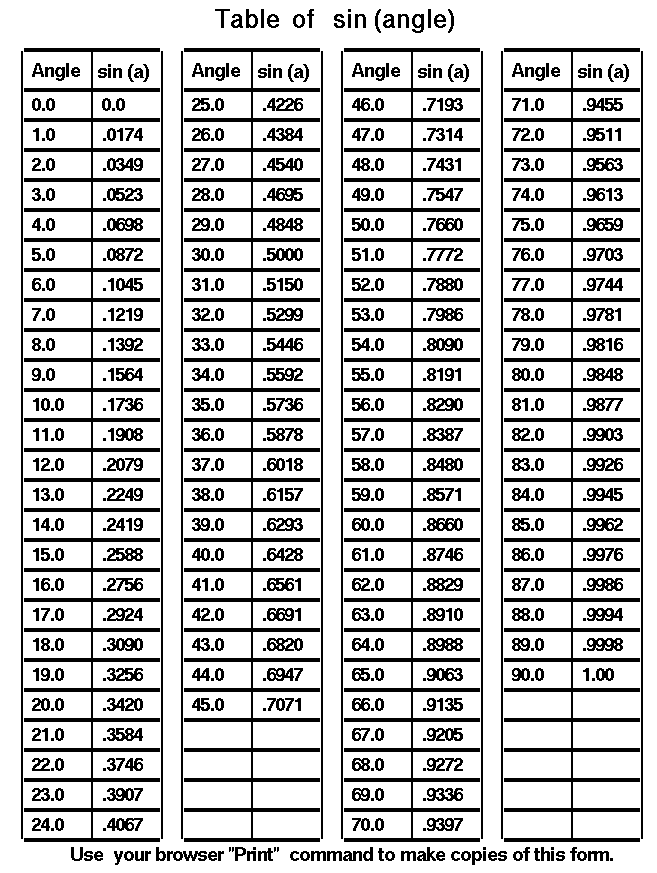JSS 3 Mathematics Second Term Week 7

Topic: SINE AND COSINE OF ANGLES

The sine (abbreviated “sin“) and cosine (“cos“) are the two most prominent trigonometric functions. All other trig functions can be expressed in terms of them. In fact, the sine and cosine functions are closely related and can be expressed in terms of each other.

Definition 1 is the simplest and most intuitive definition of the sine and cosine function. The sine definition basically says that, on a right triangle, the following measurements are related:

• the measurement of one of the non-right angles (q)
• the length of the side opposite to that angle
• the length of the triangle’s hypotenuse

Alternately, the cosine definition basically says that, on a right triangle, the following measurements are related:

• the measurement of one of the non-right angles (q)
• the length of the side adjacent to that angle
• the length of the triangle’s hypotenuse
•Furthermore, Definition I gives exact equations that describe each of these relations:

sin (q) = opposite / hypotenuse

This first equation says that if we evaluate the sine of that angle q, we will get the exact same value as if we divided the length of the side opposite to that angle by the length of the triangle’s hypotenuse. This second equation says that if we evaluate the cosine of that angle q, we will get the exact same value as if we divided the length of the side adjacent to that angle by the length of the triangle’s hypotenuse. These relations holds for any right triangle, regardless of size.

The main result is this: If we know the values of any two of the above quantities, we can use the above relation to mathematically derive the third quantity. For example, the sine function allows us to answer any of the following three questions:

“Given a right triangle, where the measurement of one of the non-right angles (q) is known and the length of the side opposite to that angle q is known, find the length of the triangle’s hypotenuse.”

“Given a right triangle, where the measurement of one of the non-right angles (q) is known and the length of the triangle’s hypotenuse is known, find the length of the sideopposite to that angle q.”

“Given a right triangle, where the length of the triangle’s hypotenuse and the length of one of the triangle’s other sides is known, find the measurement of the angle (q) oppositeto that other side.”

The cosine is similar, except that the adjacent side is used instead of the opposite side.

The functions takes the forms y = sin(q) and x = cos(q). Usually, q is an angle measurement and x and y denotes lengths.

The sine and cosine functions, like all trig functions, evaluate differently depending on the units on q, such as degrees, radians, or grads. For example, sin(90°) = 1, while sin(90)=0.89399….explaination

Both functions are trigonometric cofunctions of each other, in that function of the complementary angle, which is the “cofunction,” is equal to the other function:

sin(x) = cos(90° – x) and

cos(x) = sin(90° – x).

Furthermore, sine and cosine are mutually orthogonal.

Use of sine and cosine

Sines and cosines of angles are used to find the lengths of unknown sides in triangles. The table below shows the sines and cosines of some chosen angles.

 angle A sin A cos A 300 0.5000 0.8660 350 0.5736 0.8192 400 0.6428 0.7660 450 0.7071 0.7071 500 0.7660 0.6428 550 0.8192 0.5736 600 0.8660 0.5000

Example

In the triangle shown below, find the value of x, accurate to three decimal places.They’ve given me an angle measure and the length of the side “opposite” this angle, and have asked me for the length of the hypotenuse. The sine ratio is “opposite over hypotenuse”, so I can turn what they’ve given me into an equation:

sin(20°) = 65/x
x

I have to plug this into my calculator to get the value of xx = 190.047286…

x = 190.047

Note: If your calculator displayed a value of 71.19813587…, then check the “mode”: your calculator is set to “radians” rather than to “degrees”. You’ll learn about radians later.

For the triangle shown, find the value of y, accurate to four decimal places.They’ve given me an angle, a value for “adjacent”, and a variable for “opposite”, so I can form an equation:

tan(55.3°) = y/10
10tan(55.3°) = y

Plugging this into my calculator, I get y = 14.44183406….

y = 14.4418

Using sine and cosine tables

1. In the sine table, as angles increase from 00 to 900, their sines increase from 0 to 1.

2. In the cosine table, as angles increase from 00 to 900, their cosine decrease from 0 to 1.Solving right-angled triangles

To SOLVE A TRIANGLE means to know all three sides and all three angles.  When we know the ratios of the sides, we use the method of similar figures.  That is the method to use when solving an isosceles right triangle or a 30°-60°-90° triangle.  When we do not know the ratio numbers, then we must use the Table of ratios.  The following example illustrates how.

The general method

Example 1.   Given an acute angle and one side.   Solve the right triangle ABC if angle A is 36°, and side c is 10 cm.Solution

Since angle A is 36°, then angle B is 90° − 36° = 54°.

To find an unknown side, say a, proceed as follows:

1. Make the unknown side the numerator of a fraction, and make the known side the denominator.

Unknown/known = a/10

2. Name that function of the angle.

Unknown/known = a/10 = 360

3. Use the trigonometric Table to evaluate that function.

Unknown/known = a/10 = 360 = 0.588

4. Solve for the unknown side.

a = 10 × .588 cm = 5.88 cm

Example

Solve the triangle for side b.Unknown/known = b/a = cos 360 0.809

B = 10 X 8.09cm